# Resultant force

Calculate mathematically and graphically the resultant of a three forces with a common centre if:

F1 = 50 kN α1 = 30°
F2 = 40 kN α2 = 45°
F3 = 40 kN α3 = 25°

Correct result:

F =  113.1225 kN
f =  65.613 °

#### Solution:

$f=\frac{18{0}^{\circ }}{\pi }\cdot \mathrm{arctan}\left(y\mathrm{/}x\right)=\frac{18{0}^{\circ }}{\pi }\cdot \mathrm{arctan}\left(103.0293\mathrm{/}46.7081\right)=65.61{3}^{\circ }=6{5}^{\circ }3{6}^{\mathrm{\prime }}47\mathrm{"}$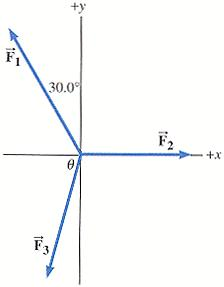We would be pleased if you find an error in the word problem, spelling mistakes, or inaccuracies and send it to us. Thank you!Tips to related online calculators
For Basic calculations in analytic geometry is helpful line slope calculator. From coordinates of two points in the plane it calculate slope, normal and parametric line equation(s), slope, directional angle, direction vector, the length of segment, intersections the coordinate axes etc.
Two vectors given by its magnitudes and by included angle can be added by our vector sum calculator.
Pythagorean theorem is the base for the right triangle calculator.

#### You need to know the following knowledge to solve this word math problem:

We encourage you to watch this tutorial video on this math problem:

## Next similar math problems:

• Side lengthsIn the triangle ABC, the height to the side a is 6cm. The height to side b is equal to 9 cm. Side "a" is 4 cm longer than side "b". Calculate the side lengths a, b.
• Outside tangents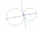Calculate the length of the line segment S1S2 if the circles k1 (S1, 8cm) and k2 (S2,4cm) touch the outside.
• Draw triangle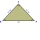Construct an isosceles triangle ABC, if AB = 7cm, the size of the angle ABC is 47°, arms | AC | = | BC |. Measure the size of the BC side in mm.
• Construct rhombusConstruct rhombus ABCD if given diagonal length | AC | = 8cm, inscribed circle radius r = 1.5cm
• Parallels and one secant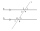There are two different parallel lines a, b and a line c that intersect the two parallel lines. Draw a circle that touches all lines at the same time.
• ConstructConstruct a triangle ABC inscribed circle has a radius r = 2 cm, the angle alpha = 50 degrees = 8 cm. Make a sketch, analysis, construction and description.
• Two chords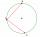There is a given circle k (center S, radius r). From point A which lies on circle k are starting two chords of length r. What angle does chords make? Draw and measure.
• Intersections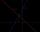Find the intersections of the function plot with coordinate axes: f (x): y = x + 3/5
• Two heights and a side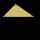Construct triangle ABC when the given side is c = 7 cm, height to side a va = 5 cm and height to side b: vb = 4 cm.
• TrianglesIvo wants to draw all the triangles whose two sides of which have a length of 4 cm and 9 cm and the length of the third side is expressed in whole centimeters. How many triangles does he have?
• Construct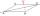Construct a rhombus ABCD, if the size of the diagonal AC is 6 cm and diagonal BD 8 cm long.
• Rhombus constructionConstruct ABCD rhombus if its diagonal AC=9 cm and side AB = 6 cm. Inscribe a circle in it touching all sides...
• Rhombus MATHConstruct a rhombus M A T H with diagonal MT=4cm, angle MAT=120°
• Rhombus EFGHConstruct the rhombus EFGH where e = 6.7cm, height to side h: vh = 5cm
• Sides od triangleSides of the triangle ABC has length 4 cm, 5 cm and 7 cm. Construct triangle A'B'C' that are similar to triangle ABC which has a circumference of 12 cm.
• DiagonalsDraw a square ABCD whose diagonals have a length of 6 cm
• Isosceles - isoscelesIt is given a triangle ABC with sides /AB/ = 3 cm /BC/ = 10 cm, and the angle ABC = 120°. Draw all points X such that true that BCX triangle is an isosceles and triangle ABX is isosceles with the base AB.